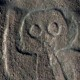## Further thoughts on “The Book of Numbers, An Infinite Book of Infinite Numbers”.

I have just posted a story based upon Jorge Luis Borges short story The Book of Sand. My story featured an infinite book of infinite numbers. While writing the story I began thinking of such a book, and what it would be like if it were to exist.

The book would have a sequence of numbers that would be infinite in length. It would include minus numbers, decimal point numbers and so on. The possibly would have this infinite sequence in all its possible permutations, every possible order it could be arranged in.

It would have every category of number (odd, even, prime, square etc) and again each set of numbers would be ordered in every possible order.

Perhaps it would also contain every possible mathematical use each individual number (sums, equations etc).

Every possible number would have to include mathematic systems that are now dead, current ones and ones yet to exist.

This is just some thoughts on what would be an impossible book. But if you are a mathematician and feel that some of this is wrong in any way do not hesitate to tell me about it. Also feel free to discuss what such a theoretical book would be like if it were to exist. Feedback is welcomed as always.